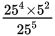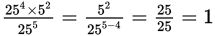# SAT Math Multiple Choice Question 877: Answer and Explanation

### Test Information

Question: 877

7. When completely simplified,has a value of:

• A. 0
• B. 1
• C. 5
• D. 25

Getting to the Answer: The power of 25 is larger in the denominator, so subtract the exponents there. Then simplify further if possible:, which is (B).